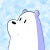# Referencing

Consider the following case

`a = [1, 2, 3]b = ab = 11print("list a:", a)print("list b:", b)`

We created a new variable `b` and assigned the value of list `a` to it, after modifying the value in list `b`, what would be the result of list…

## Hands-on Practice of Using Dataset Object

In the image recognition field, the general training set could take from GB to TB size, with each image growing bigger and bigger, there is no way to preload all images into the memory and do a model training. …

# Implement Convolutional Layer in Python

## CNN Explained

You probably have used convolutional functions from Tensorflow, Pytorch, Keras, or other deep learning frameworks. But in this article, I would like to implement the convolutional layers from scratch, which, I believe, could help one gains a deeper understanding of each component in the convolutional process.

We are going to…

# Optimization Methods in Deep Learning

## Breakdown the Fundamentals

In deep learning, generally, to approach the optimal value, gradient descent is applied to the weights, and optimization is achieved by running many many epochs with large datasets. …

# Regularization & Dropout in Deep Learning

## Code it So You Understand it

In the last post, we have coded a deep dense neural network, but to have a better and more complete neural network, we would need it to be more robust and resistant to overfitting. The commonly applied method in a deep neural network, you might have heard, are regularization and…

# Code a Deep Neural Network

## Hands on Practice of Building a Deep Neural Network in Python

In last post, we’ve built a 1-hidden layer neural network with basic functions in python. …

# One Hidden Layer NN

We will build a shallow dense neural network with one hidden layer, and the following structure is used for illustration purpose.

Before trying to understand this post, I strongly suggest you to go through my pervious implementation of logistic regression, as logistic regression can be seem as a 1-layer neural…

# Logistic Regression Step by Step Implementation

## From Theory to Practice

Say we are doing a classic prediction task, where given a input vector with \$n\$ variables:

And to predict 1 response variable \$y\$ (may be the sales of next year, the house price, etc.), the simplest form is to use a linear regression to do the prediction with the formula:

# LightGBM for Quantile Regression

## Understand Quantile Regression

For regression prediction tasks, not all time that we pursue only an absolute accurate prediction, and in fact, our prediction is always inaccurate, so instead of looking for an absolute precision, some times a prediction interval is required, in which cases we need quantile regression — that we predict an…

# Deploy a Python API on AWS

## Flask + Lambda + API Gateway

The very first idea of creating my own app and deploying it on the cloud so that everyone could use it is super exciting to me, and this is what inspired me to write this post. …## Jeremy Zhang

Hmm…I am a data scientist looking to catch up the tide…